Next: Co-Rotating Frame Up: The Three-Body Problem Previous: Jacobi Integral

Tisserand Criterion

Consider a dynamical system consisting of three gravitationally interacting point masses,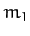,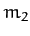, and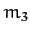. Let massrepresent the Sun, massthe planet Jupiter, and massa comet. Since the mass of a comet is very much less than that of the Sun or Jupiter, and the Sun and Jupiter are in (almost) circular orbits about their common center of mass, the dynamical system in question satisfies all of the necessary criteria to be considered an example of a restricted three-body problem.

Now, the mass of the Sun is much greater than that of Jupiter. It follows that the gravitational effect of Jupiter on the cometary orbit is negligible unless the comet makes a very close approach to Jupiter. Hence, as described in Chapter 5, before and after such an approach, the comet executes a standard elliptical orbit about the Sun with fixed orbital parameters: i.e., fixed major radius, eccentricity, and inclination to the ecliptic plane. However, in general, the orbital parameters before and after the close approach will not be the same as one another. Let us investigate further.

Now, since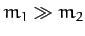, we have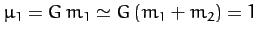, and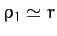. Hence, according to Equations (260) and (269), the (approximately) conserved energy (per unit mass) of the comet before and after its close approach to Jupiter is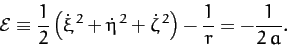(1045)

Note that the comet's orbital energy is entirely determined by its major radius,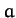. (Incidentally, we are working in units such that the major radius of Jupiter's orbit is unity.) Furthermore, the (approximately) conserved angular momentum (per unit mass) of the comet before and after its approach to Jupiter is written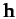, whereis directed normal to the comet's orbital plane, and, from Equations (236) and (256),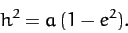(1046)

Here,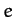is the comet's orbital eccentricity. It follows that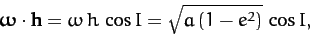(1047)

since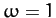in our adopted system of units. Here,is the angle of inclination of the normal to the comet's orbital plane to that of Jupiter's orbital plane.

Let,, andbe the major radius, eccentricity, and inclination angle of the cometary orbit before the close encounter with Jupiter, and let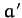,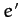, and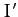be the corresponding parameters after the encounter. It follows from Equations (1044), (1045), and (1047), and the fact that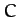is conserved during the encounter, whereas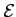and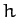are not, that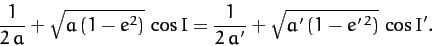(1048)

This result is known as the Tisserand criterion, and restricts the possible changes in the orbital parameters of a comet due to a close encounter with Jupiter (or any other massive planet).

The Tisserand criterion is very useful. For instance, whenever a new comet is discovered, astronomers immediately calculate its Tisserand parameter,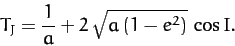(1049)

If this parameter has the same value as that of a previously observed comet then it is quite likely that the new comet is, in fact, the same comet, but that its orbital parameters have changed since it was last observed, due to a close encounter with Jupiter. Incidentally, the subscript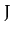in the above formula is to remind us that we are dealing with the Tisserand parameter for close encounters with Jupiter. (The parameter is, thus, evaluated in a system of units in which the major radius of Jupiter's orbit is unity). Obviously, it is also possible to calculate Tisserand parameters for close encounters with other planets.

The Tisserand criterion is also applicable to so-called gravity assists, in which a space-craft gains energy due to a close encounter with a moving planet. Such assists are often employed in missions to the outer planets to reduce the amount of fuel which the space-craft must carry in order to reach its destination. In fact, it is clear, from Equations (1045) and (1048), that a space-craft can make use of a close encounter with a moving planet to increase (or decrease) its orbital major radius, and, hence, to increase (or decrease) its total orbital energy.Next: Co-Rotating Frame Up: The Three-Body Problem Previous: Jacobi Integral
Richard Fitzpatrick 2011-03-31